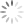# RTQ6360/61/62/63/65 60V Buck Converter Family

## Abstract

The RTQ6360/61/62/63/65 is a flexible buck converter family for wide input and output voltage range applications. It can be used with input voltages from 4.5V up to 60V and the output can be adjusted from 0.8V up to VIN. The buck converter range includes parts that can deliver currents from 0.5A up to 5A output currents. This application note explains the design criteria and describes two wide input voltage step-down designs: A low power 3.3V/0.5A output using RTQ6360GQW and a high power 24V/3A output using RTQ6363GQW. External components are calculated using the RTQ63xx Excel based design tool, and results are verified with actual measurement results.

## 1. Introduction

The RTQ6360/61/62/63/65 is a flexible asynchronous buck converter family for wide input and output voltage range applications. The parts can be used with input voltages from 4.5V up to 60V and the output can be adjusted from 0.8V up to VIN , delivering up to 5A output currents, and use peak current mode topology with external compensation for optimal flexibility. The IC design supports enhanced light load efficiency and low dropout mode operation approaching 100% duty-cycle.

 Part number Vin range Current rating Programmable Frequency range RDSON External compensation External soft-start Power good Package RTQ6360GSP 4.5 ~ 60V 0.5A 0.1 ~ 2.5MHz 170mΩ Yes No No PSOP-8 RTQ6360GQW 4.5 ~ 60V 0.5A 0.1 ~ 2.5MHz 170mΩ Yes Yes Yes DFN10L 3x3 RTQ6361GSP 4.5 ~ 60V 1.5A 0.1 ~ 2.5MHz 160mΩ Yes No No PSOP-8 RTQ6361GQW 4.5 ~ 60V 1.5A 0.1 ~ 2.5MHz 160mΩ Yes Yes Yes DFN10L 3x3 RTQ6362GSP 4.5 ~ 60V 2.5A 0.1 ~ 2.5MHz 150mΩ Yes No No PSOP-8 RTQ6362GQW 4.5 ~ 60V 2.5A 0.1 ~ 2.5MHz 150mΩ Yes Yes Yes DFN10L 3x3 RTQ6363GSP 4.5 ~ 60V 3.5A 0.1 ~ 2.5MHz 80mΩ Yes No No PSOP-8 RTQ6363GQW 4.5 ~ 60V 3.5A 0.1 ~ 2.5MHz 80mΩ Yes Yes Yes DFN10L 4x4 RTQ6365GSP 4.5 ~ 60V 5A 0.1 ~ 2.5MHz 70mΩ Yes No No PSOP-8 RTQ6365GQW 4.5 ~ 60V 5A 0.1 ~ 2.5MHz 70mΩ Yes Yes Yes DFN10L 4x4

Table 1

Chapter 2 provides an overview of the application component selection and design considerations. Chapters 3 and 4 each describe a design with specific VIN/VOUT condition from low 3.3V MCU supply to industrial supply providing 24V output.

## 2. RTQ6360/61/62/63/65 general design guidelines

The general application schematic is shown in figure 1. The Current Mode loop compensation is set via the external compensation network. The switching frequency can be programmed by the Rt resistor and the soft start time can be adjusted via the external soft-start capacitor. Due to the asynchronous topology, an external Schottky freewheel diode is needed. The output can be set via a simple resistor divider. By connecting a resistor divider from Vin to Enable, the converter Vin start and stop voltages can be precisely adjusted. The DFN package versions have Soft-start and a Power Good function which can be used for MCU reset or power sequencing.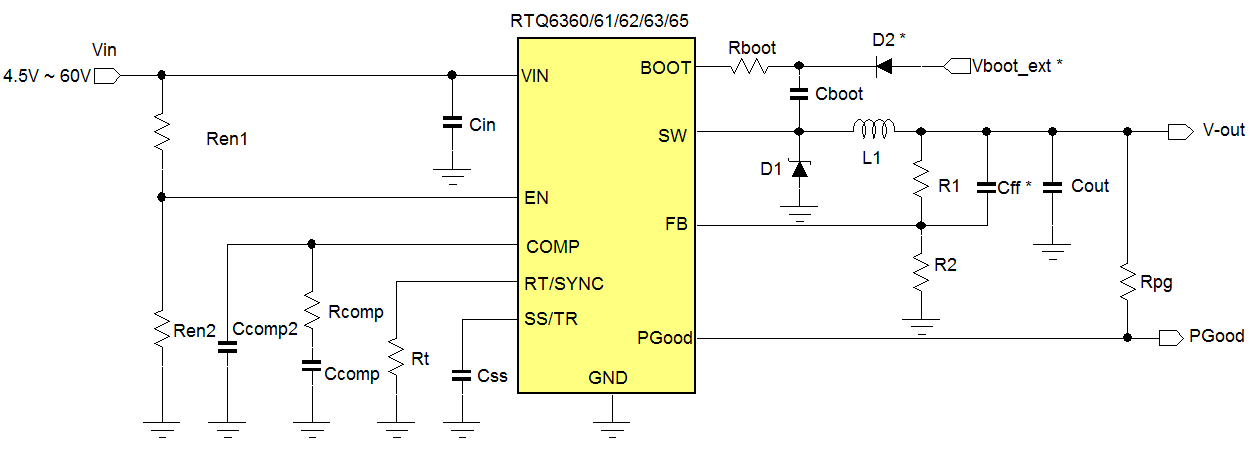Figure 1 (parts with * are optional, PGood and Softstart are only available on DFN packages)

The following guidelines can be used to calculate the various application components. For convenience, Richtek has developed an Excel calculation sheet which makes the design and component choice easy and quick. The RTQ63xx excel tool can be downloaded here.

## 3. First Example application: 3.3V/0.5A output

In this first example we will design a low current 3.3V MCU supply with a wide input voltage range from 12V to 60V, with a nominal value of 48V. This is a typical industrial MCU power supply example. We will use the RTQ63xx excel tool to show the step by step design.

The 60V maximum input voltage and the 0.5A max load current makes RTQ6360 the obvious choice. To provide a supply ready signal (Pgood) for the MCU, we will choose the DFN version RTQ6360GQW for this design. We will set the start-up voltage at 10V and shut-down voltage at 8V. The CCM output ripple should be less than 1% of the output voltage, and voltage sag during 0.2A to 0.5A load transient less than 5% of Vout. Figure 2 shows the Excel design tool input parameter section.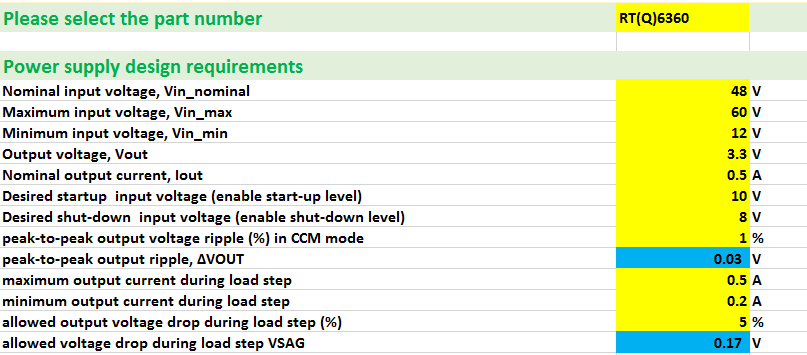Figure 2

The first step in the design is to select the switching frequency. The excel tool will calculate the maximum switching frequency based on maximum input voltage, output voltage and minimum on-time. It will also calculate the maximum switching frequency based on output short-circuit condition: This is related to the minimum duty-cycle that the converter is able to achieve in output short-circuit with frequency fold-back. For this design, we’ll set the frequency at 400kHz which will satisfy both conditions. See figure 3.Figure 3

Next step is the inductor value calculation: For this design we will set the inductor ripple current at 30% of the IC rated current. The design tool will calculate the inductor value based on this ripple current. It will also calculate the minimum required inductance to satisfy the converter slope compensation requirement. We will choose 47uH inductance, which should have a saturation current that is higher than 0.58A.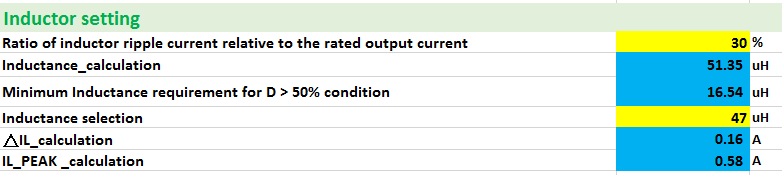Figure 4

For this design, a Würth Electronic WE-LQS 74404052470 type was choosen. Figure 5 shows the specifications.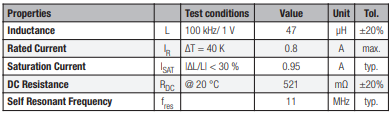Figure 5

The next step is the input capacitor selection. The design tool will calculate the required effective input capacitance to keep the peak-peak input voltage ripple lower than 1.3Vpp at worst case input voltage and output current.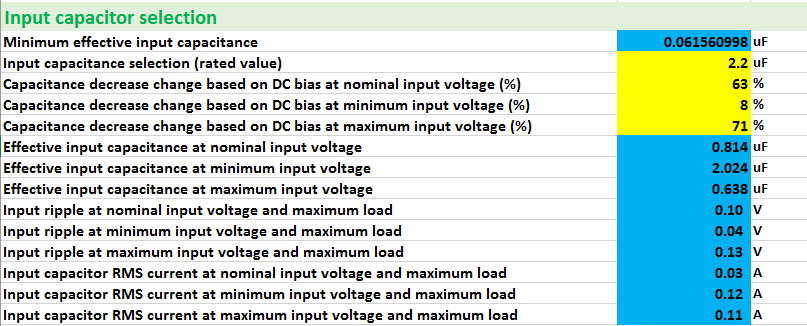Figure 6

For this design, a single 2.2µF/100V MLCC capacitor HMK316AC7225KL-TE was chosen. Since the effective capacitance highly depends on input DC voltage, we should enter the capacitance decrease rate at nominal, minimum and maximum input voltage: The capacitance decrease over DC bias can be found from the capacitance characteristics datasheet, see figure 7 left side. The design tool will then calculate the effective input capacitance and the expected input voltage ripple at each input voltage. It will also calculate the maximum RMS current in the input capacitor. You can then check the temperature rise of the capacitor at this condition (figure 7 right side). In this design the RMS ripple current is very small and hardly leads to any dissipation in the capacitor.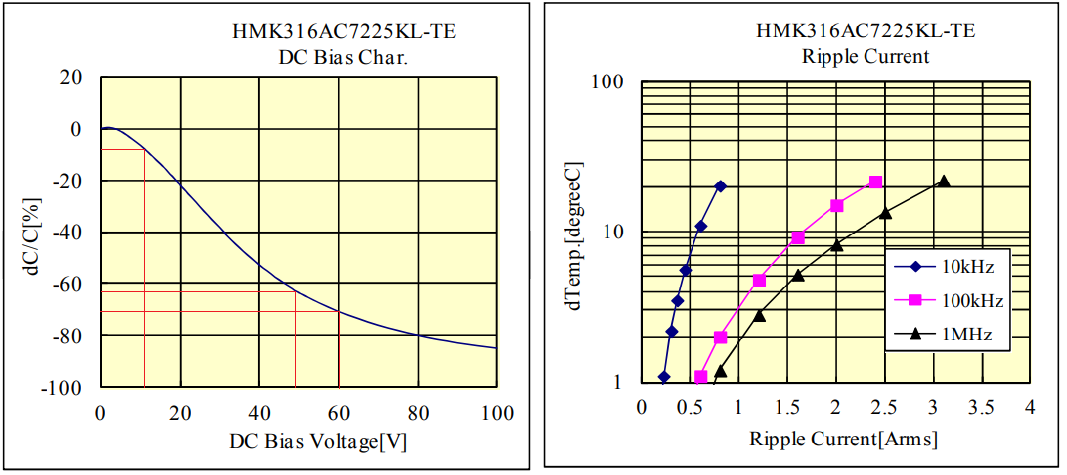Figure 7

The next step is the output capacitor selection. Its value will determine the output voltage ripple and the voltage sag during load transient at a given load step and converter control bandwidth. For this design we’ll set the control bandwidth at 10% of the switching frequency. The load step voltage sag requirement will now define the minimum required effective output capacitance value, which is 7.26μF.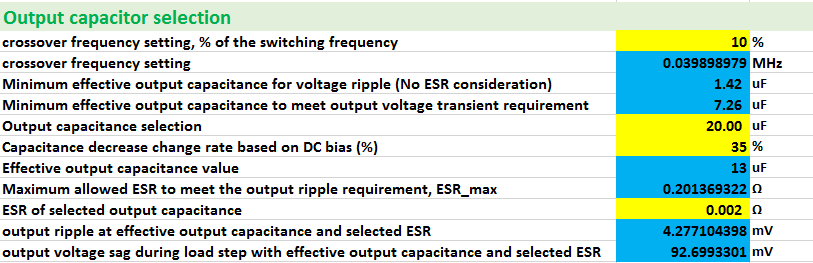Figure 8

For this design, two small size 10µF/16V X5R 0805 EMK212ABJ106KG capacitors were chosen. It is very important to include the capacitance decrease due to DC bias as well (see figure 9), as it will affect output ripple, voltage sag and converter stability. After the rated capacitance and capacitance % decrease at 3.3V DC bias has been entered in the design tool, it will calculate the effective total output capacitance, the minimum required ESR to meet the output ripple specification, and the actual output ripple and voltage sag.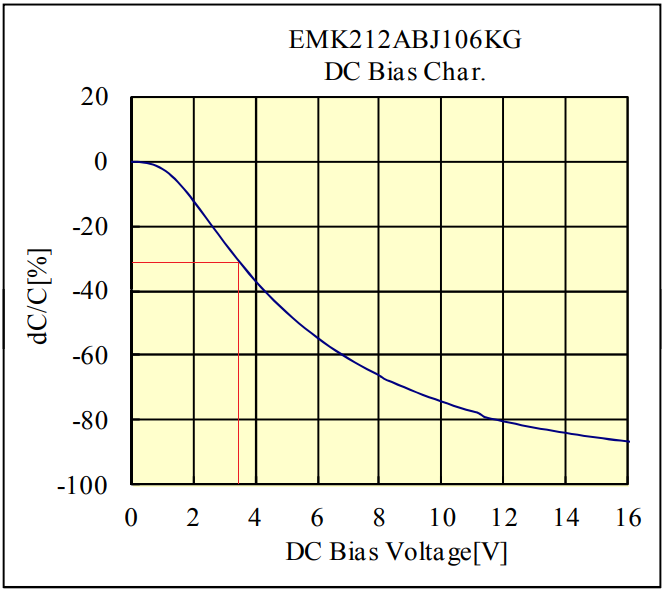Figure 9

Next step is the Freewheel diode selection. The RTQ63xx series require an external Schottky diode to provide a current path for the inductor current when the high-side MOSFET is off. The forward drop of the Schottky diode should be low, to reduce the power loss in the diode, but also to avoid current flow in the IC internal small low-side MOSFET that is used for boot strap capacitor re-charge in light load. (see figure 10). This means that the forward voltage drop of the Schottky diode at maximum load current should be low compared to the body diode of the IC internal low side MOSFET. For Schottky diodes this is normally not a problem.Figure 10

For this low current supply, switching losses will be a major part of the total losses. The Schottky diode capacitance and reverse recovery effects should be minimized. Finally, since this supply can work with input voltage up to 60V, the Schottky diode voltage rating should be sufficient, and reverse leakage current at maximum reverse voltage and maximum ambient temperature should be checked as well.

For this design a 60V/1A PMEG6010ER Schottky diode was chosen. The important parameters are shown in figure 11.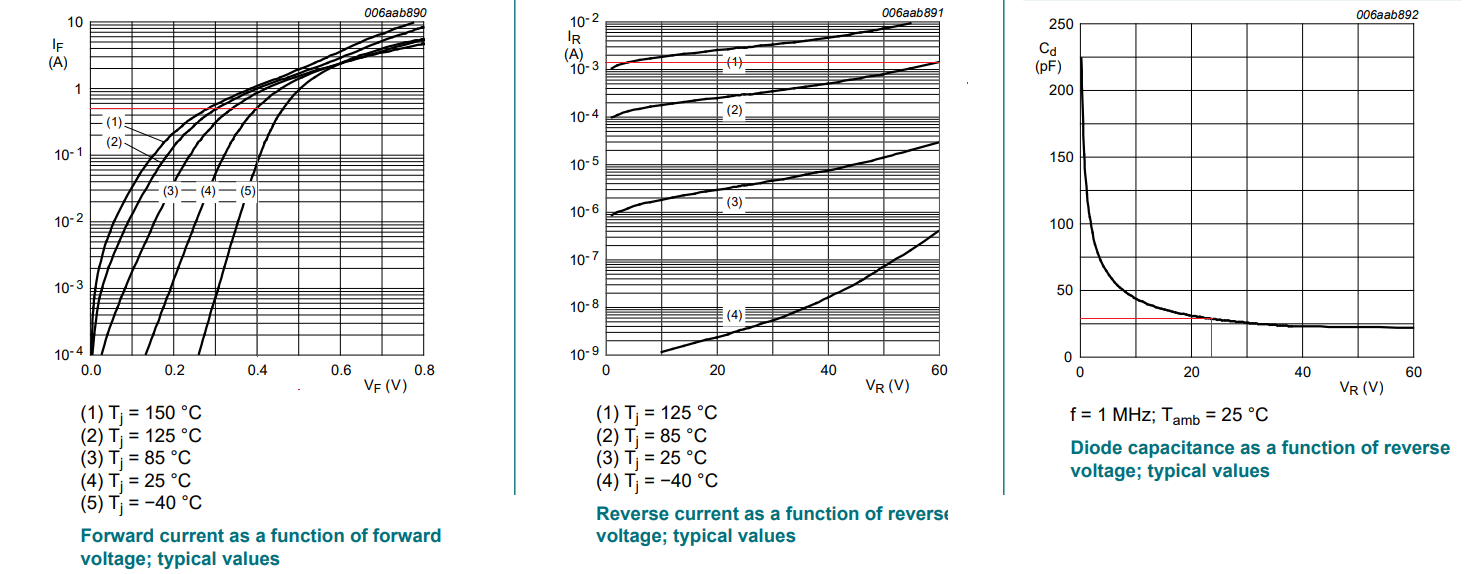Figure 11

The PMEG6010ER forward voltage at 25°C and 0.5A is 0.4V and the diode worst case forward voltage values will not come close to the limit values as shown in the RTQ6360 datasheet. At 85°C ambient and 60V reverse voltage, the reverse leakage current is 1.3mA. The power loss due to leakage current is D*VIN*ILEAKAGE = 0.055*60*0.0013 = 4mW, which will not have much impact on the converter efficiency and will not cause thermal runaway. The Schottky diode junction capacitance is highly dependent on diode reverse voltage. This capacitance influences the IC internal high-side MOSFET switching losses, so lower capacitance Schottky diodes will reduce the switching losses, especially at higher input voltages.

The calculation of the feedback resistors is straightforward: the designer inputs the low-side feedback resistor and the tool calculates the high-side resistor.Figure 12

The calculation of the compensation resistor RCOMP is based on the previously entered converter bandwidth, the effective output capacitance and the IC parameters. CCOMP is then calculated to set the compensation zero to coincide with the converter load pole. For low ESR ceramic output capacitors, the CCOMP2 capacitors is calculated to set the compensator pole at half the switching frequency. Please note that RTQ6360 COMP pin internally has 5.7pF to ground, so the external CCOMP2 value can be 5.7pF lower than the calculated one. See figure 13.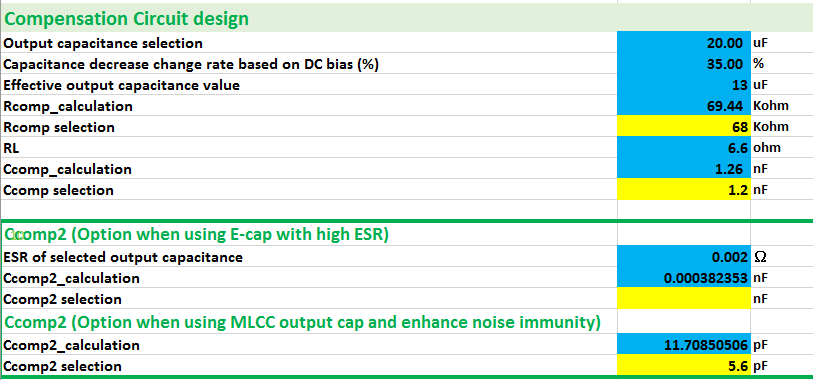Figure 13

The design can be finalized with the calculation of the Enable resistor divider and Soft-start capacitor: With the entered VIN start and stop voltages, the design tool calculates the recommended upper REN1 resistor, and after entering the actual value, it calculates the second REN2 resistor and shows the actual start and stop levels based on the actual resistor values used.

The soft-start time for the 3.3V converter is not critical and we choose an arbitrary 3msec soft-start time, which requires a 10nF soft-start capacitor. The PGOOD pin is pulled up with a 4.7kΩ to VOUT. See figure 14.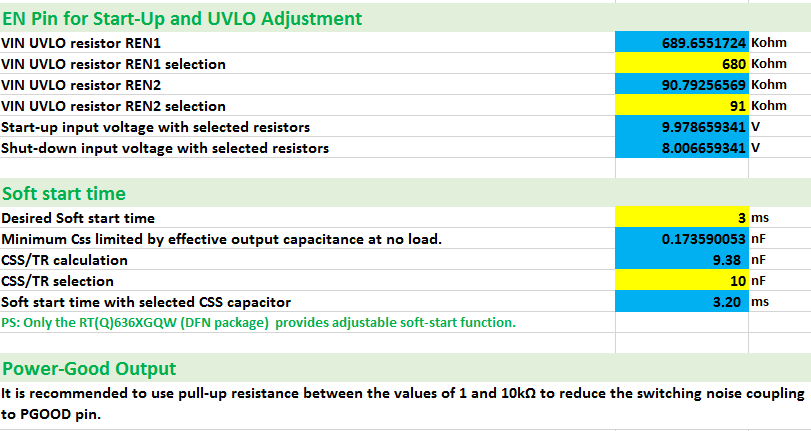Figure 14

The complete application circuit and a PCB layout example is shown in figure 15.Figure 15

The circuit was built on the RTQ6360GQW evaluation board, and the all performance items were measured and compared with calculated values: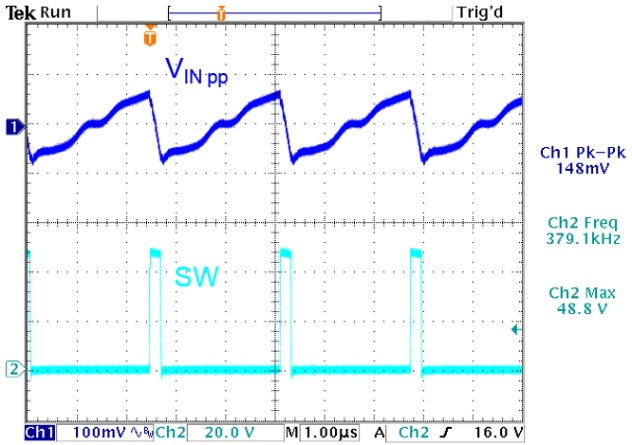Measurement of input ripple at VIN = 48V, VOUT = 3.3V, 0.5A load Calculated value: 100mVpp, measured value: 148mVpp

Figure 16

 Output ripple in PSM mode VIN = 48V, VOUT = 3.3V, 1mA load Output ripple in CCM mode VIN = 48V, VOUT = 3.3V, 0.5A load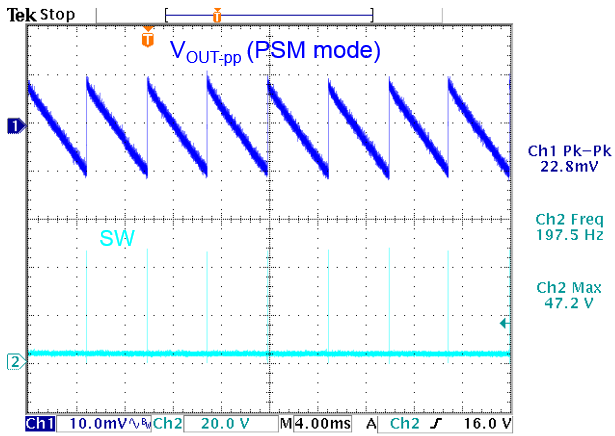Figure 17Measured value: 22.8mVpp Calculated value: 4.3mVpp, measured value: 8.8mVpp

The converter voltage sag during load step was measured by using the Richtek Fast Load transient Tool.

 VIN = 48V, 0.2A ~ 0.5A fast load step VIN = 24V, 0.2A ~ 0.5A fast load step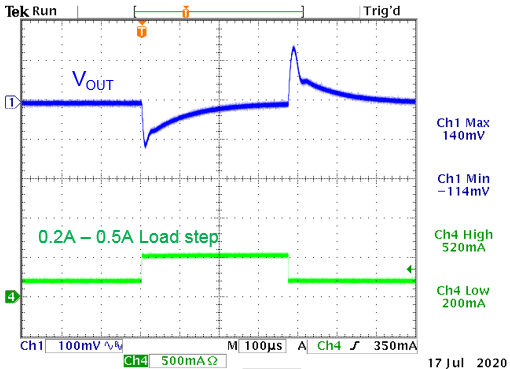Figure 18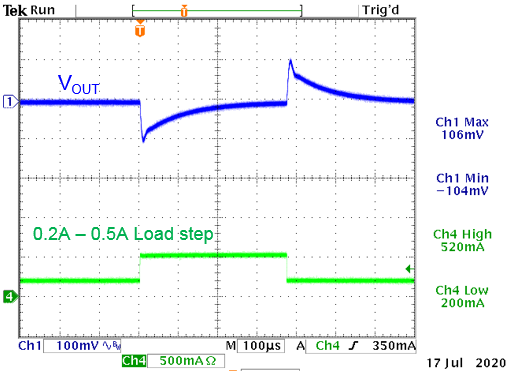Calculated value:92mV; measured value: 114mV Calculated value: 92mV, measured value: 104mV

The step load response at 48V input voltage in figure 18 left side is a bit different from the 24V input condition (figure 18 right side), especially the overshoot when load goes from 0.5A to 0.2A. During sudden load drop, the converter will try to quickly reduce its on-time. At 48V input, the converter is already working close to its minimum on time, so the headroom in on-time reduction is limited, hence resulting in larger overshoot. The step load in both cases shows a small ring, which points to lower phase margin.

A gain-phase measurement (figure 19) shows this more clearly:Figure 19 VIN = 48V, Vout = 3.3V / 0.5A load: Bandwidth = 28kHz, Phase margin 46 dgs

The gain-phase Bode plot shows that the loop phase drops quite fast above the crossover frequency. This can be slightly improved by removing the CCOMP2 capacitor of 5.6pF:

Figure 20 shows the gain-phase measurement result when CCOMP2 is removed: The phase margin is slightly better.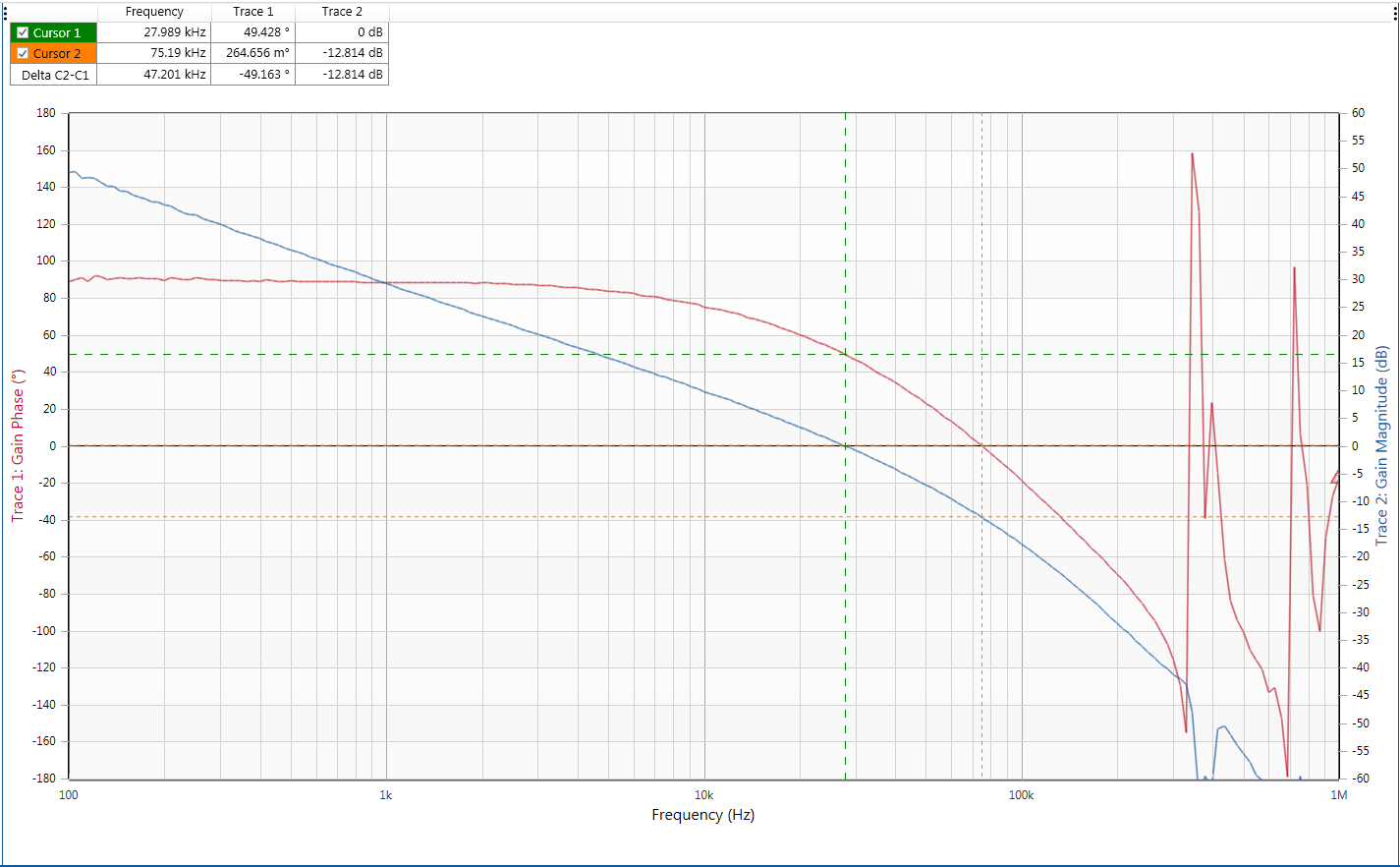Figure 20 VIN = 48V, Vout = 3.3V/0.5A load and removed CCOMP2 capacitor: Bandwidth = 28kHz, Phase margin 49 dgs

If better phase margin is required, it is recommended to set the converter bandwidth frequency setting percentage at a lower value (e.g. 7% instead of 10% of FSW) at the expense of slightly larger voltage sag during load steps and re-calculating the RCOMP, CCOMP and CCOMP2 values.

The converter start-up from VIN and Shut-down from VIN was also measured. The measurement results match the calculated values quite well. The small hysteresis step in the Enable signal can be seen when it passes the threshold. See figure 21.

 Start-up at rising VIN: Shut-down at falling VIN:Figure 21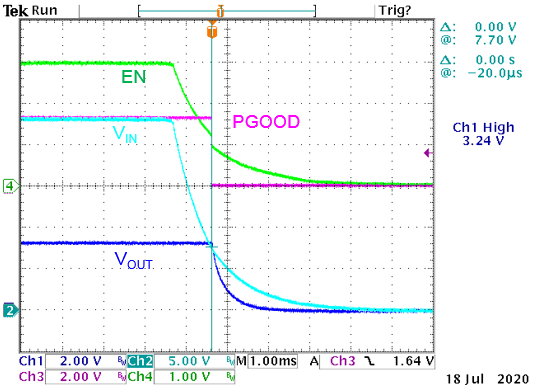VSTART calculated: 10V; VSTART measured: 10.2V VSTOP calculated: 8V; VSTOP measured: 7.7V

The converter efficiency was also measured at different input voltages: Figure 22 shows that the efficiency is highly dependent on the input voltage.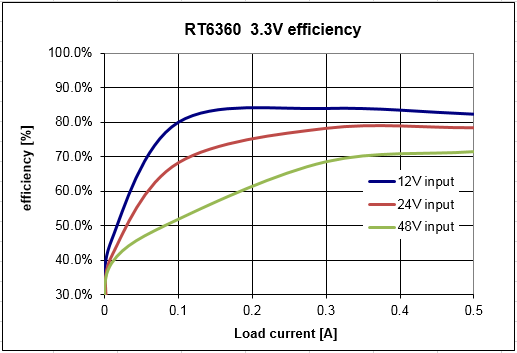Since efficiency = POUT / (POUT + PLOSS) it is useful to analyze the power losses of the converter.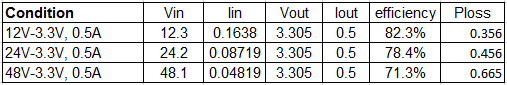As can be seen, the total power losses at 48V input are almost double of the losses in 12V input condition, which is mainly caused by the higher switching losses at higher input voltage.

Figure 22

## 4. Second Example application: 24V/3A (72W) output

In this second example we will design a 24V/3A converter running from an industrial 48V supply. This is a high power design and correct component selection is quite important.

For the IC selection we can either choose the RTQ6363 (3.5A version) or the RTQ6365 (5A) version). The RDSON of the 5A version is slightly better than the 3.5A version, but the 5A version has higher current limit, which means that an inductor with larger saturation current must be used. In this example, we will select the RTQ6363, and choose the DFN 4x4-package version RTQ6363GQW, which has better thermal resistance compared to the PSOP-8 package.

We will use the RTQ63xx excel design tool again to calculate the component values.

We will set the start-up voltage at 35V and shut-down voltage at 28V, which ensures clean start-up of the power supply. CCM output ripple should be less than 1% of the output voltage, and voltage sag during 1A to 3A load transient should be less than 5% of Vout. Figure 23 shows the Excel design tool input parameter section.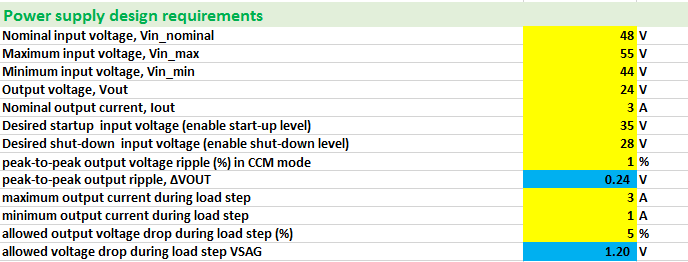Figure 23

The first step in the design is to select the switching frequency. The excel tool will calculate the maximum switching frequency based on maximum input voltage, output voltage and minimum on-time, as well as the maximum switching frequency based on output short-circuit condition. As the duty-cycle for this 48V ~ 24V design lies around 50%, the minimum on-time will not easily be reached, allowing higher switching frequencies. But it is not wise to choose high switching frequencies for 48V input supplies as the switching losses will increase considerably. To minimize the switching losses, we will set the frequency at 300kHz. See figure 24.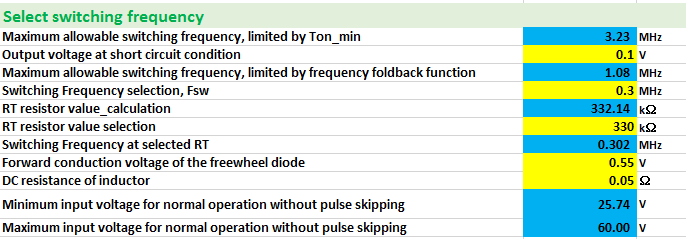Figure 24

Next step is the inductor value calculation: With the selecting 30% ripple current, the design tool will calculate the inductor value based on this ripple current to be 38µH. The minimum required inductance to satisfy the converter slope compensation requirement is 27µH. We will choose 47µH inductance, which will give an 850mApp current ripple. The inductor saturation current should be higher than 3.4A and the RTQ6363GQW 5.5A peak current limit should also be considered when choosing the inductor saturation current.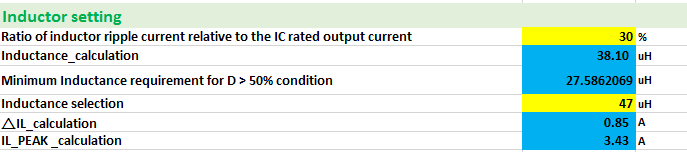Figure 25

For this design, a Würth Electronic WE-PD 7447709470 type was choosen which has 4.5A saturation current. Figure 26 shows the specifications. Slight saturation during over-current situations is allowed.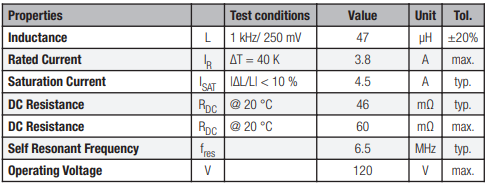Figure 26

The next step is the input capacitor selection. The design tool will calculate the required effective input capacitance to keep the peak-peak input voltage ripple lower than 1.3Vpp at worst case input voltage and output current.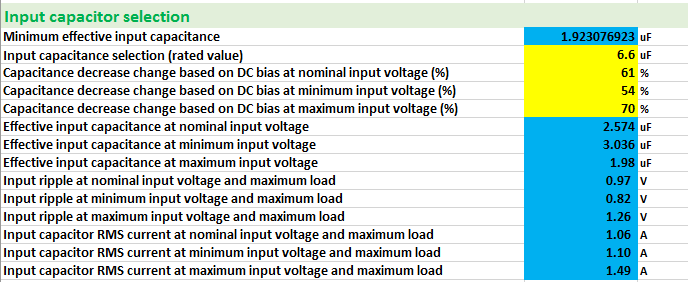Figure 27

For this design, we will use three pieces 2.2µF/100V MLCC capacitors HMK316AC7225KL-TE in parallel to get sufficient capacitance at high input voltage. After we enter the capacitance decrease rate at nominal, minimum and maximum input voltage from the capacitor datasheet graph, the design tool will calculate the effective input capacitance and the expected input voltage ripple at each input voltage. It will also calculate the maximum RMS current in the input capacitor. As we use three capacitors in parallel, each capacitor will see 1/3 of the calculated RMS current so worst case RMS current in each capacitor is 0.5A. As you can see in figure 28 right side, the temperature rise of the capacitor at this condition is still small.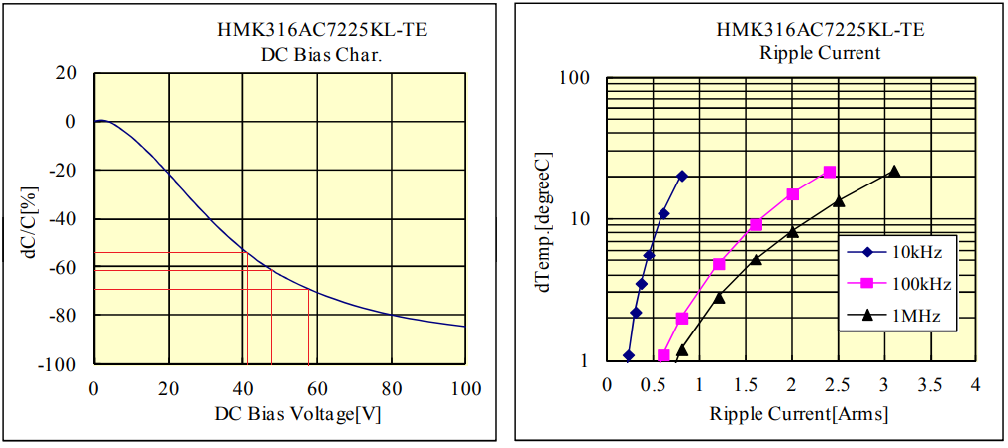Figure 28

The next step is the output capacitor selection. Its value will determine the output voltage ripple and the voltage sag during load transient at a given load step and converter control bandwidth. For the high voltage and high power design moderate control bandwidth is recommended. We start with a control bandwidth of 10% of the switching frequency. The load step voltage sag requirement will now define the minimum required effective output capacitance value, which is 8.85μF.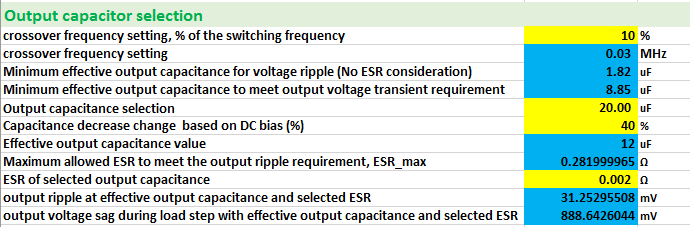Figure 29

For this design, two 1210 size 10µF/50V X7S UMR325AC7106KM capacitors in parallel were chosen. It is very important to include the capacitance decrease due to DC bias as well (see figure 30), to ensure correct compensation calculation results. This capacitor type drops around 40% at 24Vdc. After the rated capacitance and 40% decrease at 24V has been entered, the design tool will calculate the effective total output capacitance, the minimum required ESR to meet the output ripple specification, and the actual output ripple and voltage sag.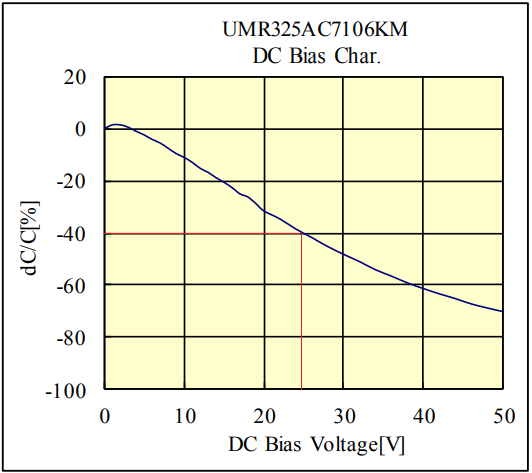Figure 30

Next step is the freewheel diode selection. The total power dissipation in this design will be high, resulting in higher operation temperatures of the power components. It is therefore important to select parts that will operate reliably at high temperatures. With larger Schottky diodes, reverse leakage currents can be a critical parameter at high temperatures, and for this design we selected a Trench type 60V/5A PMEG060T050ELPE Schottky which exhibits lower reverse leakage current compared to normal planar Schottky diodes (1). The important parameters are shown in figure 31.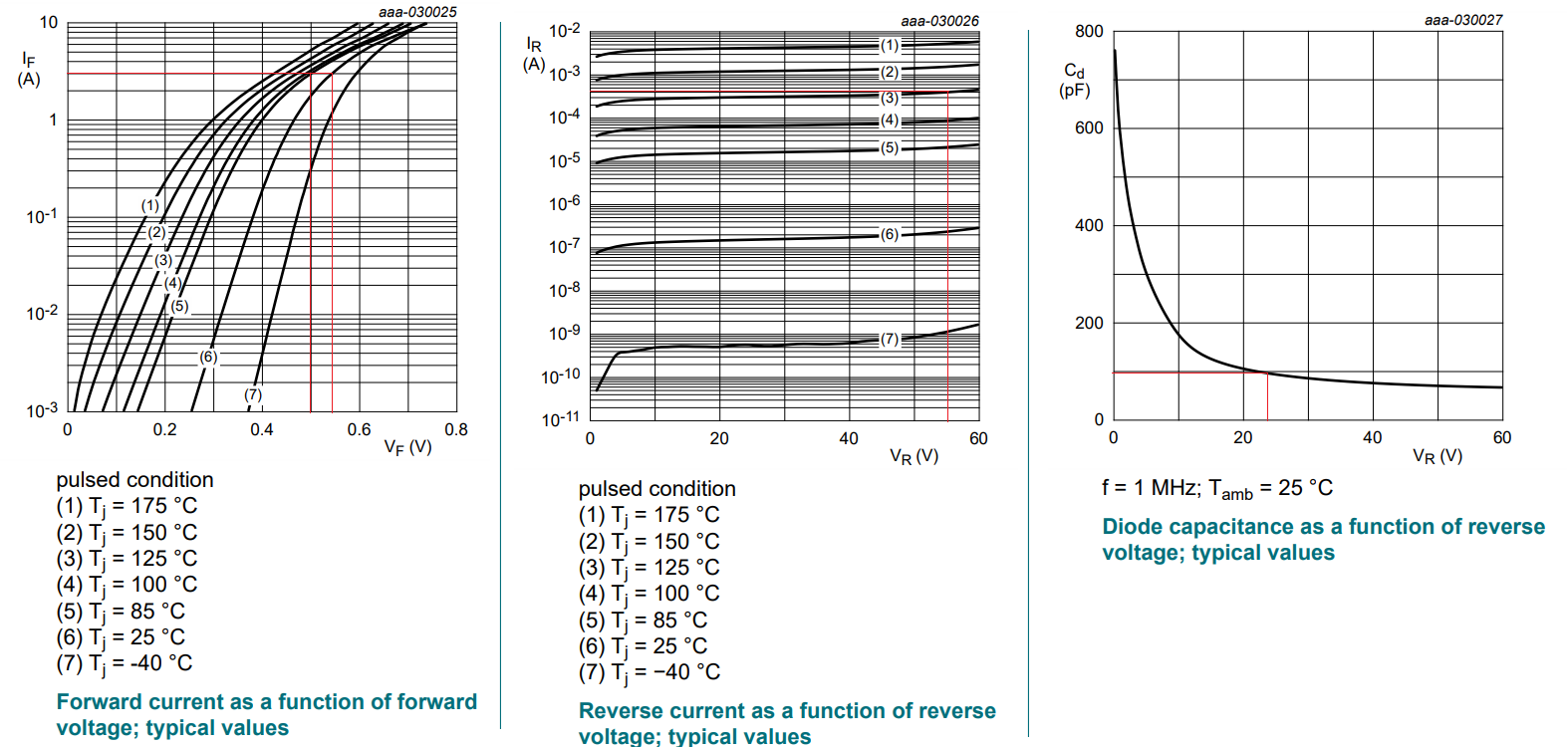Figure 31

At 125°C ambient and 55V reverse voltage, the reverse leakage current is 0.4mA. The power loss due to leakage current is very low: D*VIN*ILEAKAGE = 0.45*55*0.0004 = 9.9mW.

The junction capacitance is highly dependent on diode reverse voltage. As this capacitance influences the IC internal high side MOSFET switching losses, Schottky diodes with lower capacitance can help reduce switching losses, although the reverse recovery charge of the diode will also play a role (1).

The PMEG060T050ELPE forward voltage at 25°C and 3A is 0.54V and the diode worst case forward voltage values will not come close to the limit values as shown in the RTQ6363 datasheet, so there is no risk of current flow in the body diode of the RTQ6363 small low side MOSFET. See figure 32.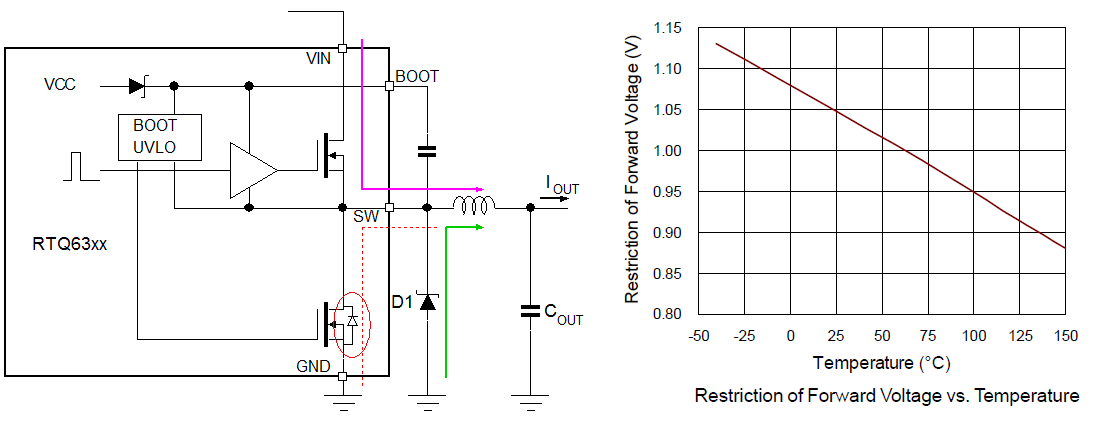Figure 32

The calculation of the feedback resistors is straightforward: the designer inputs the low-side feedback resistor and the tool calculates the high-side resistor.Figure 33

The calculation of the compensation resistor RCOMP is based on the previously entered converter bandwidth, the effective output capacitance and the IC parameters. CCOMP is then calculated to set the compensation zero to coincide with the converter load pole. For low ESR ceramic output capacitors, the CCOMP2 capacitors is calculated to set the compensator pole at half the switching frequency. Please note that RTQ6363 COMP pin internally has 26pF to ground, so the external CCOMP2 value can be 26pF lower than the calculated one. See figure 34.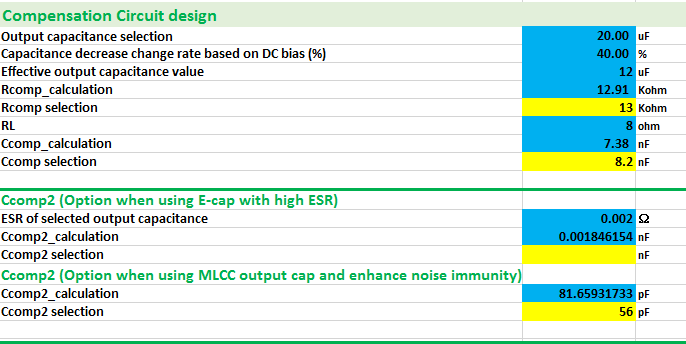Figure 34

The 48V to 24V design duty-cycle does not reach 65%, so the external bootstrap charge circuit is not needed.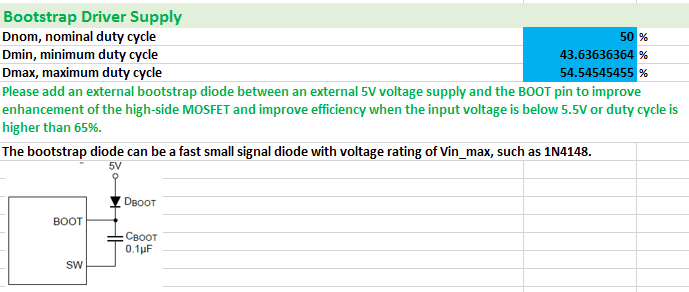Figure 35

Finally the Enable resistor divider and Soft-start capacitor can be calculated. As the effective output capacitance (12µF) is relatively low the inrush current during soft-start is also low. We choose the 3msec soft-start time again, which requires a 10nF soft-start capacitor. See figure 36.

The PGOOD pin in this example is pulled up with a 10kΩ to VOUT, but for power sequencing it could be pulled up to an external low voltage supply or apply a resistor divider from VOUT.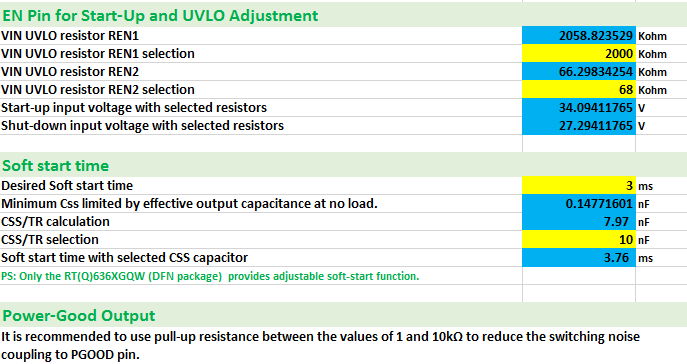Figure 36

The complete application circuit and a PCB layout example is shown in figure 37.Figure 37

The circuit was built on the RTQ6363GQW evaluation board, and the all performance items were measured and compared with calculated values:

 Input ripple measurement at VIN = 48V, VOUT = 24V, 3A load Input ripple measurement at VIN = 55V, VOUT = 24V, 3A load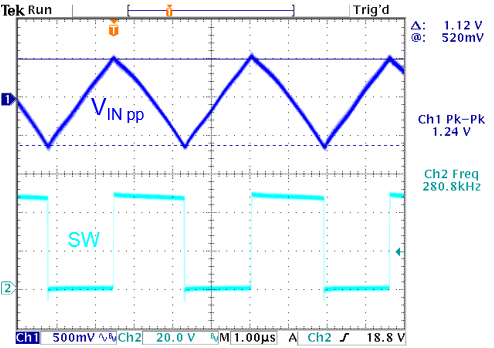Figure 38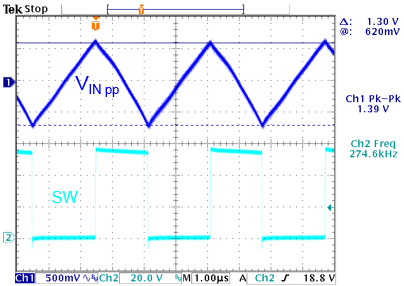Calculated value: 0.97Vpp, measured value: 1.12Vpp Calculated value: 1.26Vpp, measured value: 1.30Vpp

 Output ripple in PSM mode VIN = 48V, VOUT = 24V, 1mA load Output ripple in CCM mode VIN = 48V, VOUT = 24V, 3A loadFigure 39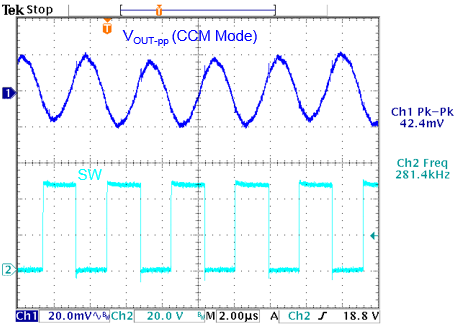Measured value: 9.6mVpp Calculated value: 32mVpp, measured value: 42mVpp

The converter voltage sag during load step was measured by using the Richtek Fast Load transient Tool.

 VIN = 48V, 1A ~ 3A fast load step VIN = 48V, 1A ~ 3A fast load step estimate bandwidth: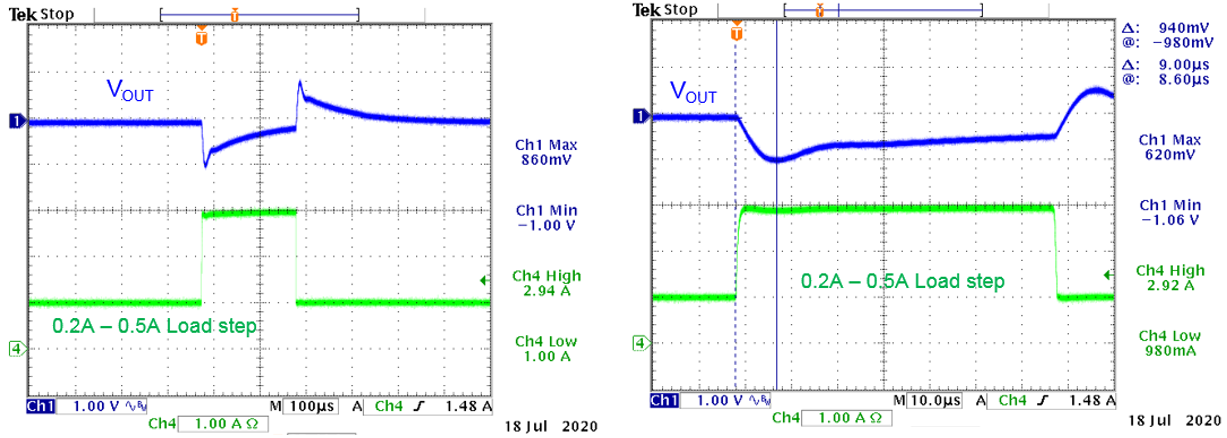Figure 40 Vsag calculated value:0.88V; measured value: 1.0V Response time = 9μsec. BW ≈ 0.3/9μsec = 33kHz

The step load shows a small ring, which points to lower phase margin. A gain-phase measurement (figure 41) shows this more clearly:Figure 41

At VIN = 48V, and 24V with 3A load, the bandwidth shows 35kHz and phase margin is 48dgs. Gain margin is 11dB.

But when measuring gain-phase at 1A load the phase margin became worse: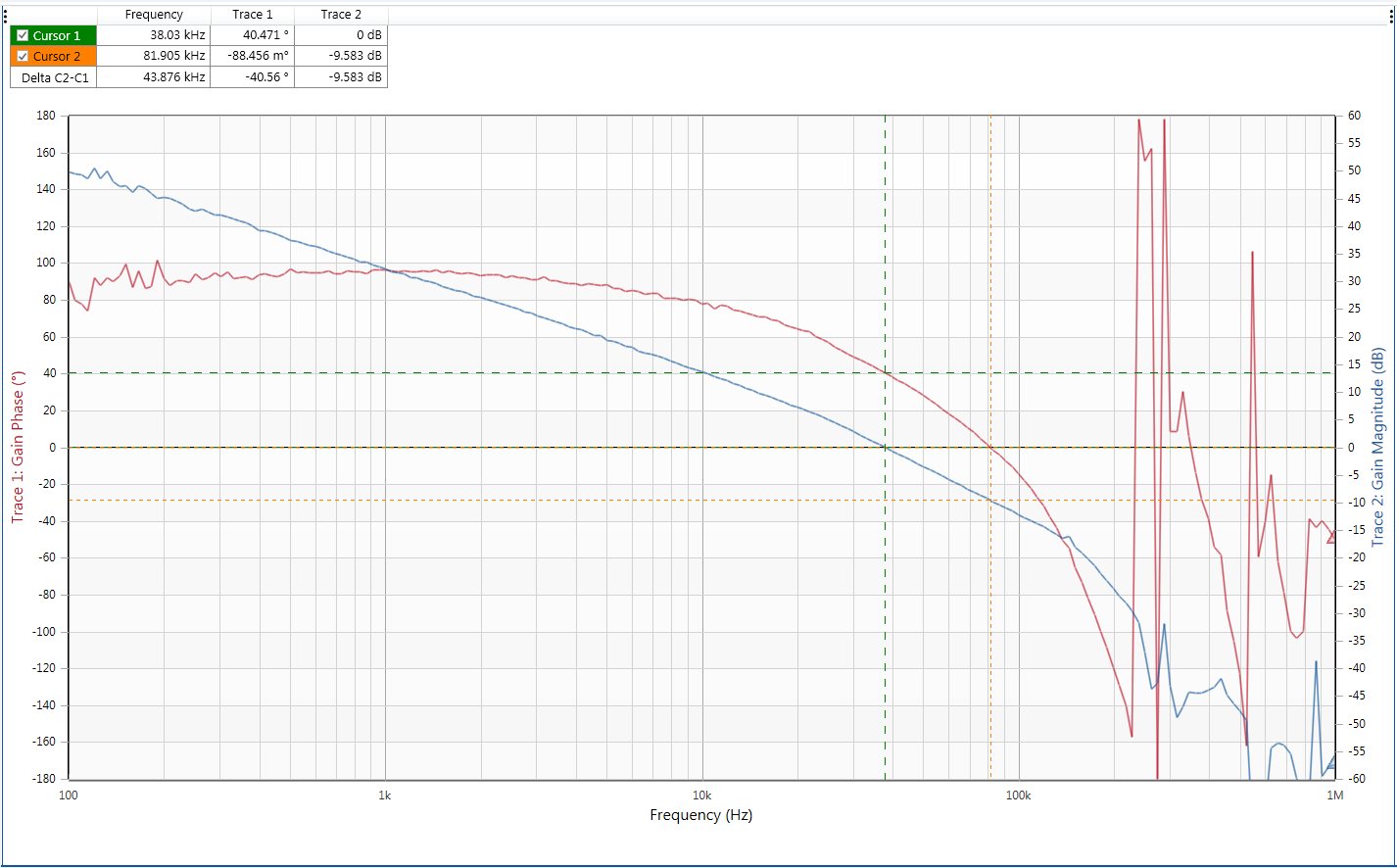Figure 42

At VIN = 48V, and 24V with 1A load, the bandwidth shows 38kHz and phase margin is 41dgs. Gain margin is 9.6dB.

This phase margin is not sufficient and should be improved. Adding a small feed-forward capacitor CFF can boost the phase but it will also increase the bandwidth and lower the gain margin:

Figure 43 shows the gain-phase measurement result when 22pF CFF is added in parallel with the upper feedback resistor.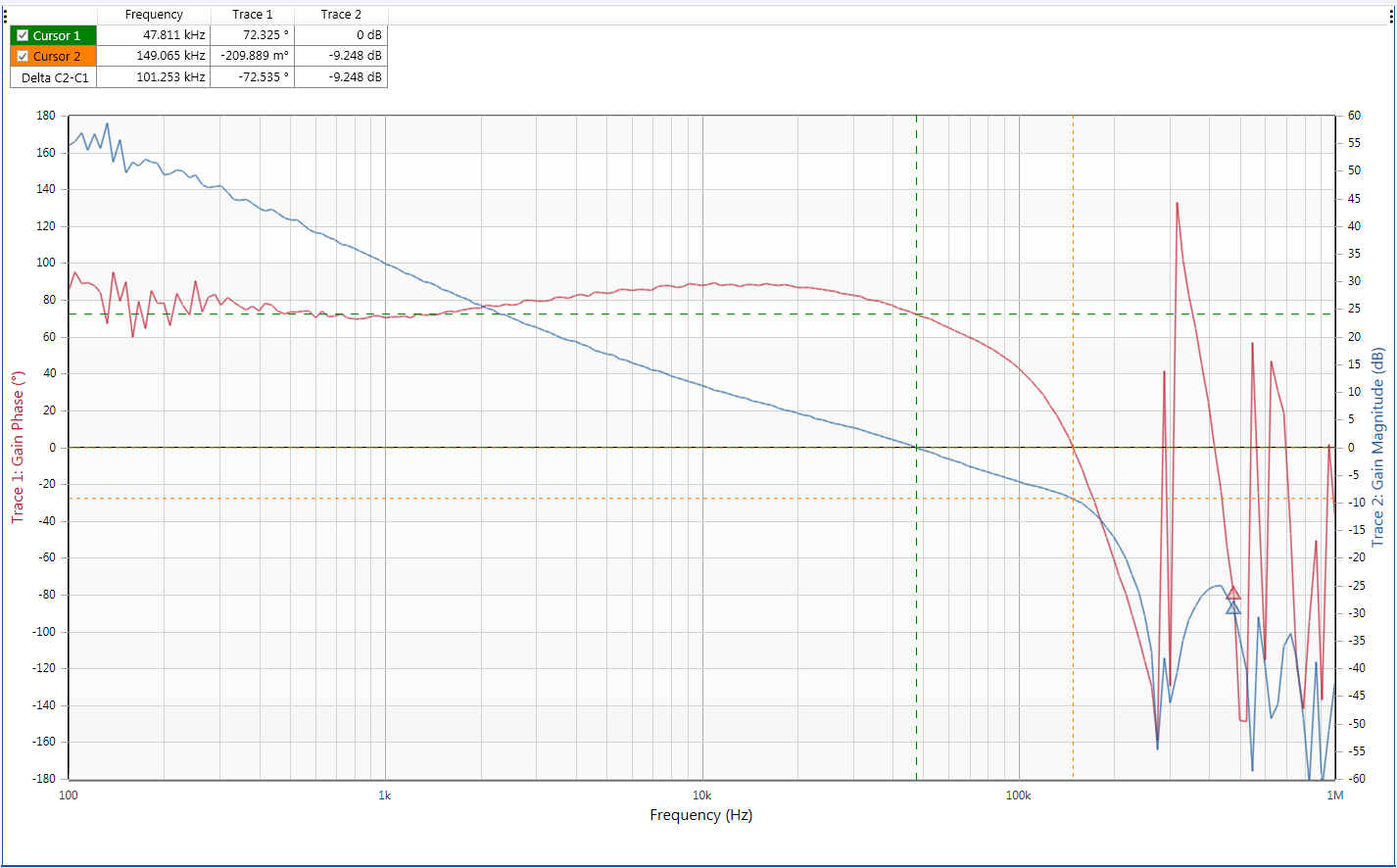Figure 43

At VIN = 48V, and 24V with 1A load and added 22pF CFF, the bandwidth shows 48kHz and phase margin is 72dgs. But the Gain margin is only 9.2dB which is rather low.

A more stable design can be achieved by reducing the converter bandwidth; Figure 44 shows the gain-phase measurement when using a BW setting of 6% of the switching frequency. RCOMP = 7.5k, CCOMP = 12nF and CCOMP2 = 100pF, and no CFF.Figure 44

At VIN = 48V, and 24V with 1A load and compensation for 6% BW setting, the bandwidth shows 27kHz and phase margin is 58dgs. Gain margin is 13dB. This is a more stable design.

Due to the lower bandwidth setting, the load step voltage sag will become larger, but the step load response is without ring.

 VIN = 48V, 0.2A ~ 0.5A fast load step VIN = 48V, 1A ~ 3A fast load step estimate bandwidth: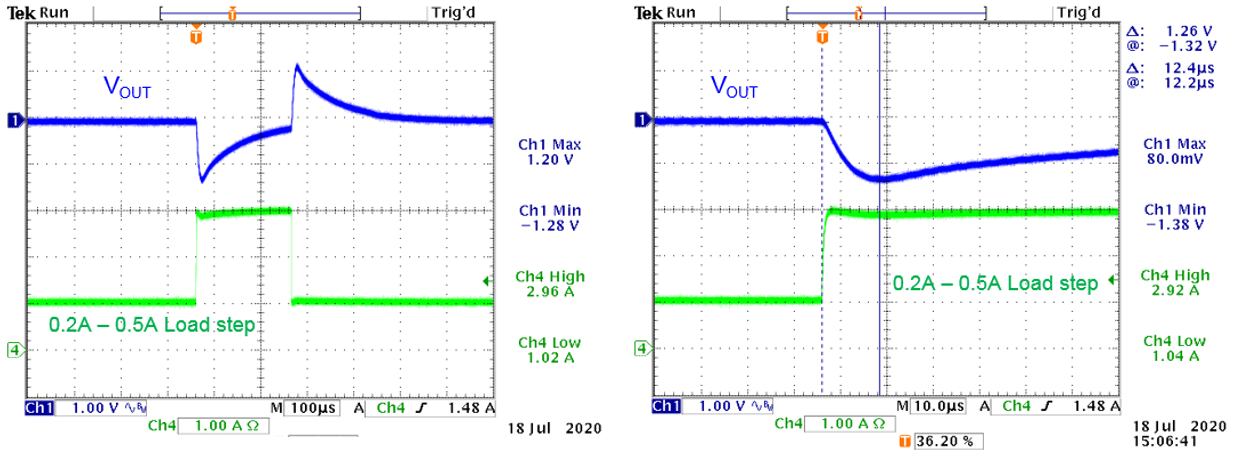Figure 45 Calculated value:1.4V; measured value: 1.3V Response time = 12.4μsec. BW ≈ 0.3/12.4μsec = 24kHz

The converter start-up from VIN and Shut-down from VIN were also measured. The small hysteresis step in the Enable signal can be seen when it passes the threshold. See figure 46.

 Start-up at rising VIN: Shut-down at falling VIN:Figure 46 VSTART calculated: 34V; VSTART measured: 31.4V VSTOP calculated: 27.2V; VSTOP measured: 27V

The converter efficiency and power loss was also measured, see figure 47.Figure 47

The total power loss at 3A load is 3.6W: Please note that the IC, Schottky diode and inductor all dissipate power so the heat is distributed as well. The IC however dissipates the majority of power and its thermal pad should be connected to sufficient copper on top layer and with thermal vias to inner layers and bottom layer.

## 5. Schottky diode selection: Reverse leakage current

In the original 48V – 24V/3A design, we had selected a special Trench type Schottky diode, which has greatly reduced reverse leakage current compared to standard Planar Schottky diodes (1). With the Trench type Schottky diode, the power loss due to leakage is extremely small. To show the differences between these two types of diodes in this high power design, we will also test the Nexperia PMEG060V050EPDZ Planar Schottky diode. The reverse leakage current graphs of the Trench and Planar type Schottky diodes are shown in figure 48.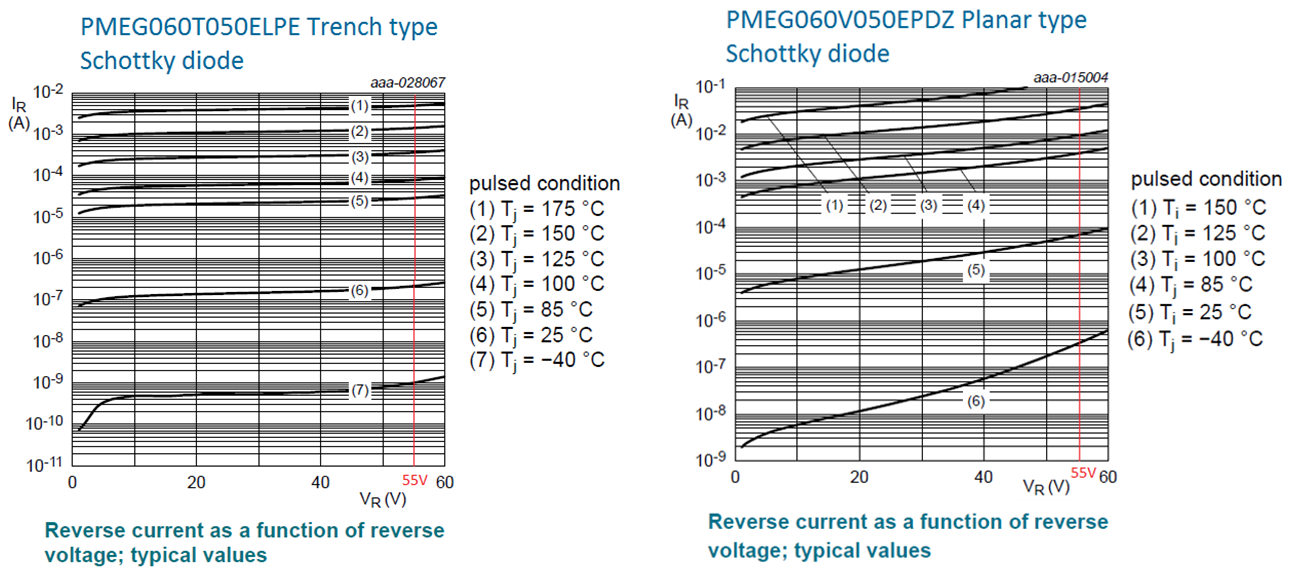Figure 48 At maximum 55V input voltage and 100°C, the reverse leakage current is 80μA. Reverse leakage Power loss = D*VIN*ILEAKAGE = 0.5*55V*80μA = 2mW At maximum 55V input voltage and 100°C, the reverse leakage current is 10mA. Reverse leakage Power loss = D*VIN*ILEAKAGE = 0.5*55V*10mA = 275mW At 125°C, the reverse leakage current is 350μA. Reverse leakage Power loss = 0.5*55V*350μA = 10mW At 125°C, the reverse leakage current is 34mA. Reverse leakage Power loss = 0.5*55V*34mA = 0.935W

The calculations show that the reverse leakage current of a standard planar Schottky diodes can result in higher power loss in the diode at high temperatures.

This was verified by measurements: The two diodes were tested in the same 24V/3A application with 55V input, and the ambient temperature was gradually increased. Initially the Planar Schottky diode has slightly less power loss as its forward voltage is lower than the Trench type Schottky. But above 105°C, the power loss of the Planar Schottky increases rapidly, and at around 115°C it rises by itself and the diode fails at around 125°C due to excessive dissipation. See figure 49.

Figure 49

This example shows that Schottky diode reverse leakage current can be a critical parameter for application reliability.

## 6. Conclusion

The RTQ63xx family can be used successfully in a wide range of step-down converter applications. The RTQ63xx excel design tool makes it easy to calculate the external components, and the measured performance matches the calculated values quite well. It is recommended to check the stability by means of gain-phase analysis although a fast step load test can also be used to quickly check stability aspects. For higher duty-cycle and high input voltage applications, the Schottky diode capacitance, and reverse leakage current are important parameters that must be considered.

References:

(1)Benchmarking of a Novel SiGe Diode Technology for the Usage in High Frequency 48V/12V Converter applications by A. Aneissi, M.Meissner, K.F. Hoffmann, R. Behtash, J. Fisher, S. FahlbuschTable of Content
Related Parts
TitlePart NoDocument
42VIN, 0.5A, Asynchronous Step-Down Converter with Low Quiescent Current RT6340
42VIN, 1.5A, Asynchronous Step-Down Converter with Low Quiescent Current RT6341
42VIN, 2.5A, Asynchronous Step-Down Converter with Low Quiescent Current RT6342
42VIN, 3.5A, Asynchronous Step-Down Converter with Low Quiescent Current RT6343
42VIN, 5A, Asynchronous Step-Down Converter with Low Quiescent Current RT6345
42VIN, 0.5A, Asynchronous Step-Down Converter with Low Quiescent Current RTQ6340
42VIN, 2.5A, Asynchronous Step-Down Converter with Low Quiescent Current RTQ6342
42VIN, 3.5A, Asynchronous Step-Down Converter with Low Quiescent Current RTQ6343
42VIN, 5A, Asynchronous Step-Down Converter with Low Quiescent Current RTQ6345
60VIN, 0.5A, Asynchronous Step-Down Converter with Low Quiescent Current RT6360
60VIN, 1.5A, Asynchronous Step-Down Converter with Low Quiescent Current RT6361
60VIN, 2.5A, Asynchronous Step-Down Converter with Low Quiescent Current RT6362
60VIN, 3.5A, Asynchronous Step-Down Converter with Low Quiescent Current RT6363
60VIN, 5A, Asynchronous Step-Down Converter with Low Quiescent Current RT6365
60VIN, 0.5A, Asynchronous Step-Down Converter with Low Quiescent Current RTQ6360
60VIN, 1.5A, Asynchronous Step-Down Converter with Low Quiescent Current RTQ6361
60VIN, 2.5A, Asynchronous Step-Down Converter with Low Quiescent Current RTQ6362
60VIN, 3.5A, Asynchronous Step-Down Converter with Low Quiescent Current RTQ6363
60VIN, 5A, Asynchronous Step-Down Converter with Low Quiescent Current RTQ6365
TOP